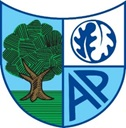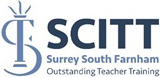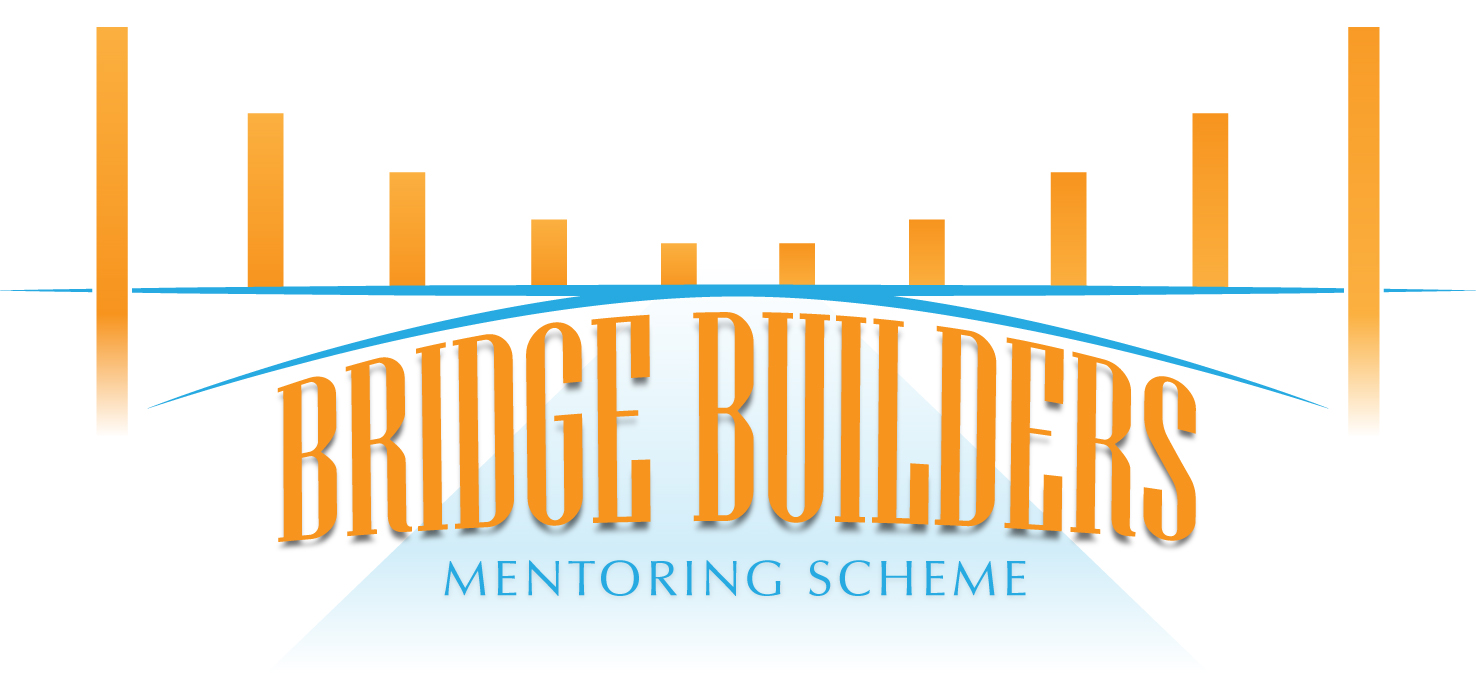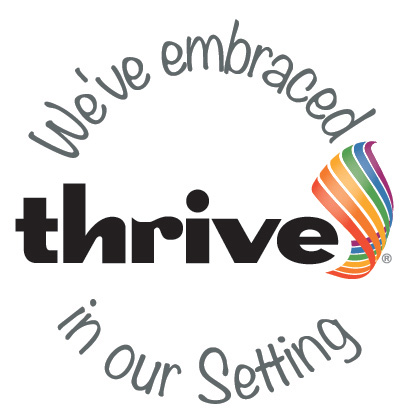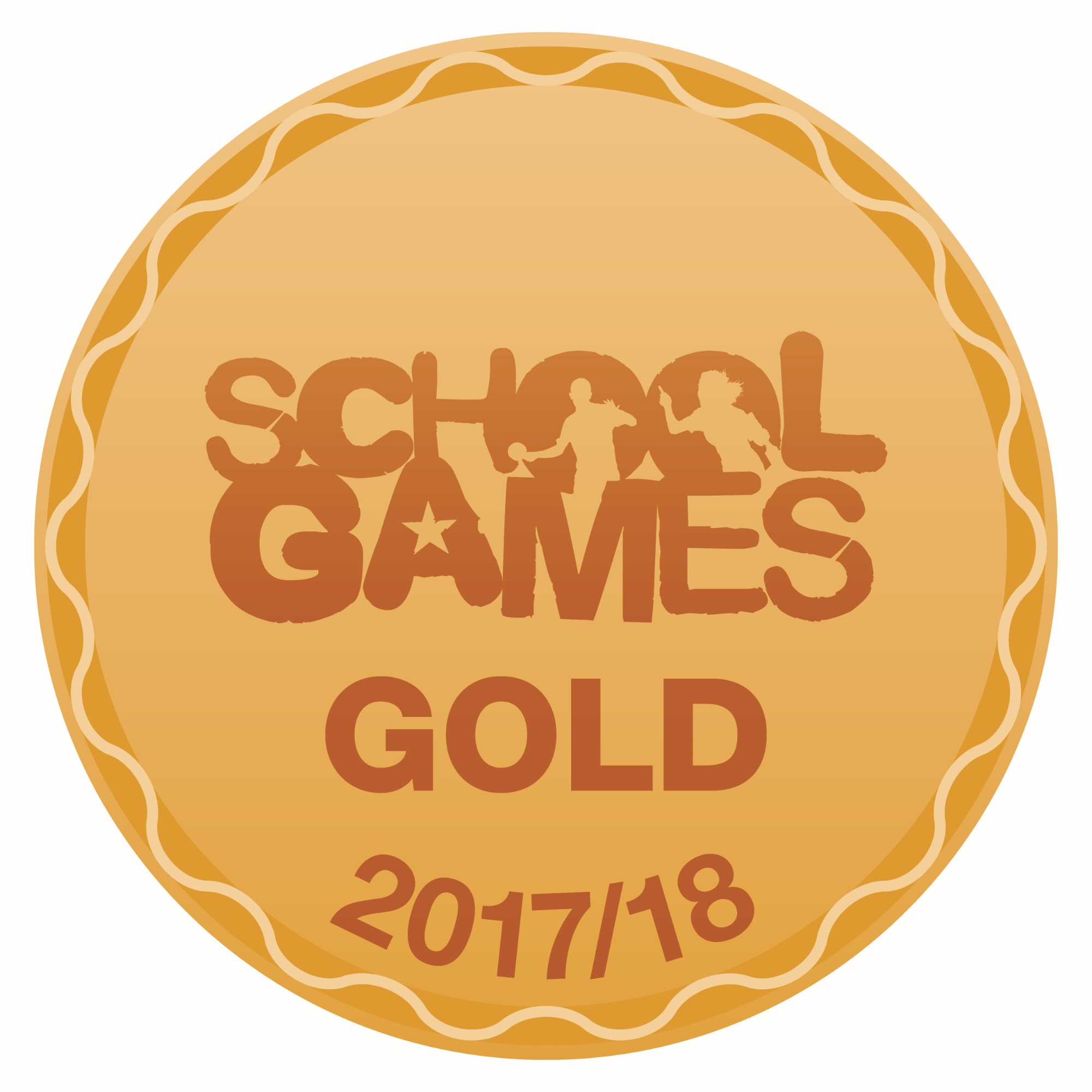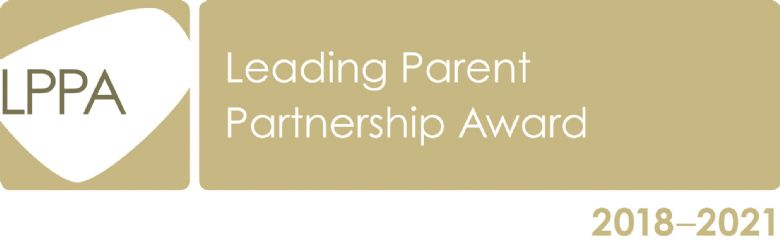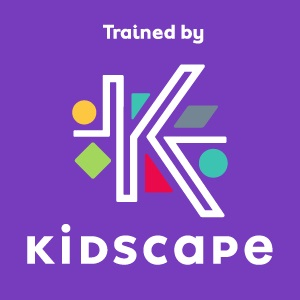# Mathematics

## RATIONALE

Please find a link to the National Curriculum Programmes of Study for Mathematics: National Curriculum mathematics, programme of study

Mathematics teaches us how to make sense of the world around us through developing a child’s ability to calculate, to reason and to solve problems. It enables children to understand and appreciate relationships and pattern in both number and space in their everyday lives.

Through our mathematics lessons at APPS it is our aim to develop:

• Enjoyment and enthusiasm for mathematics and an awareness of the fascination of mathematics.
• Confidence and fluency in mathematical knowledge, concepts and skills.
• An ability to use and apply mathematics across the curriculum and in real life.
• An ability to solve problems, by applying their skills to a variety of routine and non-routine problems. To reason, to think logically and to work systematically and accurately.
• An ability to reason mathematically and explain relationships using mathematical language.
• An understanding of mathematics through a process of enquiry and experiment.
• Initiative and an ability to work both independently and in cooperation with others.

The teaching and learning of mathematics at Ashford Park

At APPS we use a variety of teaching and learning styles in mathematics lessons. Our principal aim is to develop children’s knowledge, skills and understanding in mathematics. We do this through daily 'Early Bird Maths' and mathematics lessons where children are given opportunities for:

• Practical activities and mathematical games
• Problem solving
• Individual, group and whole class discussions and activities
• A range of methods of calculating e.g. mental, pencil and paper and using a calculator
• Working with computers as a mathematical tool
• Using a wide range of support resources e.g. number squares, digit cards and number lines
• Using and applying their learning in everyday situations

Maths games to play at home

Learning guides and resources:

Maths Support Booklet - EYFS

Maths Support Booklet – 1

Maths Support Booklet – 2

Maths Support Booklet – 3

Maths Support Booklet – 4

Maths Support Booklet – 5

Maths Support Booklet – 6

Progression In Written Division

Progression In Written Multiplication

Progression In Written Subtraction

Please find the following websites to REALLY extend your mathematical thinking - ENJOY!